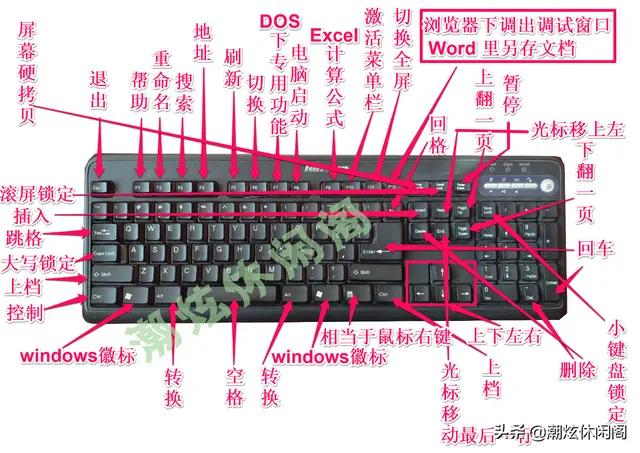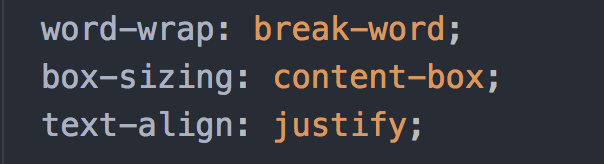• 解决两端对齐导致标点符号位于行首问题 最近在公司做一个自适应网站，需求要求文本两端对齐，那肯定是 text-align: justify; 上一篇为了兼容火狐与IE加了 word-break: break-all; 但是后来发现有几款手机会出现...
解决两端对齐导致标点符号位于行首问题
最近在公司做一个自适应网站，需求要求文本两端对齐，那肯定是
 text-align: justify;

上一篇为了兼容火狐与IE加了
 word-break: break-all;

但是后来发现有几款手机会出现标点符号出现在行首的情况还有一个链接也被分开分成两行了，这种情况肯定是不可以的，一开始是修改文字来改。但是这种改法肯定会引起其他尺寸的手机显示异常，不是根本的解决方案。后来发现了可以解决这个问题的代码就是：
  text-align: justify;
word-break: normal;

这个同样可以兼容火狐、IE8以上。IE8以下没有测试不知道可行不。


展开全文html css
• 键盘是最常用的输入设备，通过键盘可以将汉字、英文字母、数字、标点符号等输入到计算机中，从而向计算机发出命令、输入数据。那么键盘分为几个功能区？各键的功能是什么呢？组合键又有什么功能呢？下面就分别予以...键盘是最常用的输入设备，通过键盘可以将汉字、英文字母、数字、标点符号等输入到计算机中，从而向计算机发出命令、输入数据。那么键盘分为几个功能区？各键的功能是什么呢？组合键又有什么功能呢？下面就分别予以说明 (以win10 OS 、联想台式机键盘等为例)。一、键盘分区一般的标准键盘分为功能区、主键盘区、控制键区和数字键区。二、键盘各键功能1.功能键区ESC：取消光标所在行F1：系统默认帮助信息F2：选中文件及文件夹重命名F3：资源管理下搜索文件功能F4：显示"我的电脑(win xp)"或"计算机(win7)"或"此电脑(win10)"和"Windows 资源管理器"中的"地址"栏、浏览器地址栏F5：刷新键F6：在窗口或桌面上循环切换屏幕元素。F7：DoS下专用功能F8：电脑启动，BIOS启动项设置F9：Excel 中计算公式F10：激活菜单栏(当可用时)F11：全屏模式F12：浏览器下调出调试窗口、Word 另存文档2.控制键区Num Lock指示灯：小键盘数字锁定，亮起表示数字输入状态Caps Lock指示灯：大小写锁定，亮起表示输入大写Scoll Lock指示灯：滚动锁定指示灯，亮起表示页面滚动状态PriScSyRp：屏幕拷贝键(截全屏键)Scoll Lock：滚动锁定，在需要翻页的时候，按下看看，有奇效PauseBreak：暂停键Insert：插入字符开关键Home：行首键，光标移至行首位置PageUp：向上翻页键盘Delele：字符删除键，当前光标后面的字符删除End：行尾键，光标移至行尾位置PageDn：向下翻页键盘四个方向键：上下左右移动光标3.数字键区NumLocK：锁定数字小键盘Del：字符删除键，当前光标后面的字符删除Enter:回车键Home：行首键，光标移至行首位置End：行尾键，光标移至行尾位置PgUp：向上翻页键盘PgDn：向下翻页键盘Lns: Insert：插入字符开关键主键盘区Tab：跳转功能Backspace (有的键盘为向左的箭头)：回格键或删除键CapsLock：字母大小写切换Shift：按住Shift键，在选择文件可以连续选择Ctrl：按住Ctrl键，在选择文件可以不连续多个选择Alt：关闭当前的菜单Enter：回车键，换行作用Windows：单按显示或隐藏开始菜单空格键右侧(Windows键与Ctrl键之间)页面上一个斜箭头那个键：同鼠标右键功能三、组合键1. Windows组合键Windows + F：查找所有文件Windows + Ctrl+ F：查找计算机Windows+E：打开资源管理Windows+R：打开运行对话框Windows+D：显示或隐藏桌面Windows+M：最小化所有窗口Windows+Shift+M:撤消最小化所有窗口Windows+L：锁屏(切换用户)Windows + P：选择演示显示模式Windows+U：打开设置显示窗口Windows+ F1: 显示"帮助"Window+Ctrl+D：创建新的虚拟桌面Window+Ctrl+F4：关闭当前虚拟桌面Windows+Ctrl+左箭头键：转到左侧的虚拟桌面Windows+Ctrl+右箭头键：转到右侧的虚拟桌面Windows+左箭头键：将当前窗口移到左侧。Windows+右箭头键：将当前窗口移到右侧。Windows+向上箭头键：将当前窗口移到到顶部Windows+向下箭头键：将当前窗口移到到底部Windows+Tab：打开一个新的任务视图界面，显示此虚拟桌面上的所有当前窗口Windows + pause break：显示"系统属性"对话框Windows + F：打开"反馈中心"Windows + K ：打开"连接"快速操作Windows+ A： 新通知Windows+Alt+D ：显示和隐藏桌面上的日期和时间Windows + X ：打开"快速链接"菜单Windows+H ：麦克风Windows+I：打开"Windows设置"窗口Windows+O ：锁定设备方向Windows+S： 打开快速搜索Windows+T： 在任务栏上循环切换应用Windows + V：打开剪贴板Windows + 逗号 (,): 临时快速查看桌面Windows + Shift + V: 以相反的顺序循环切换通知Windows + Z: 显示在以全屏模式呈现的应用中可用的命令Windows + Ctrl + F: 搜索电脑(如果你位于网络中)Windows + 数字: 打开桌面，并启动固定到任务栏的位于该数字所表示位置的应用。如果该应用已在运行，则切换到该应用Windows + Shift + 数字: 打开桌面，并启动固定到任务栏的位于该数字所表示位置的应用的新实例Windows + Ctrl + 数字:打开桌面，并切换到固定到任务栏的位于该数字所表示位置的应用的最后一个活动窗口Windows + Alt + 数字: 打开桌面，并打开固定到任务栏的位于该数字所表示位置的应用的跳转列表Windows + Ctrl + Shift + 数字: 打开桌面，并以管理员身份打开位于任务栏上给定位置的应用的新实例Windows + Home: 最小化除活动桌面窗口以外的所有窗口(在第二道笔划时还原所有窗口)Windows+ Shift + 向上键: 将桌面窗口拉伸到屏幕的顶部和底部Windows+ Shift + 向下键: 在垂直方向上还原/最小化活动桌面窗口，同时保持宽度不变Windows+ Shift + 向左键或向右键: 将桌面中的应用或窗口从一个监视器移动到另一个监视器Windows + 空格键: 切换输入语言和键盘布局Windows+ Ctrl + 空格键:更改为以前选择的输入Windows + 加号 (+) 或减号 (-): 使用"放大镜"放大或缩小Windows + Esc :退出"放大镜"2. Ctrl L组合键Ctrl+A：全选Ctrl+B：整理 收藏夹Ctrl+C：复制Ctrl+D：删除/添加收藏 (在Word中是修改选定字符格式,在IE及傲游中是保存为收藏)Ctrl+E： 搜索助理 在Word中是段落居中Ctrl+F： 查找Ctrl+ G： 在Word中是定位Ctrl+H ：历史记录 在Word中是替换Ctrl+I：收藏夹Ctrl+J：IE7.0里的源Ctrl+ K 在Word中是插入链接Ctrl+L＝Ctrl+O：打开Ctrl+ M： 网页搜索框中的确定(等于回车)在Word中是调整整段缩进Ctrl + N：新窗口Ctrl + P ：打印 在Word中是等于Ctrl+Shift+F12Ctrl + R ：刷新 在Word中可使段落右对齐Ctrl + S ：保存Ctrl+TIE7以上打开新选项卡Ctrl+U：在Word中是给所选内容加下划线Ctrl+V：粘贴Ctrl+W：关闭当前窗口Ctrl+X：剪切Ctrl+Y：恢复上一步操作Ctrl+Z：撤消上一步操作Ctrl+ 向右方向键：将插入点移动到下一个单词的起始处Ctrl+ 向左方向键：将插入点移动到前一个单词的起始处Ctrl+ 向下方向键：将插入点移动到下一段落的起始处Ctrl+ 向上方向键：将插入点移动到前一段落的起始处Ctrl+ Shift +方向键：突出显示一块文本Ctrl+ Esc ：显示"开始"菜单Ctrl+F4： 在允许同时打开多个文档的程序中关闭当前文档Ctrl+Tab： 在选项卡之间向前移动。Ctrl+Shift +Tab： 在选项卡之间向后移动ctrl+鼠标左键： 可以临时允许弹出窗口程序Ctrl+Home ：当前窗口或文档的最上端Ctrl+End ：当前窗口或文档的最下端Ctrl + Shift + 左键单击某程序图标： 以管理员身份运行3.Alt组合键Alt+PrtScSysRq：截当前窗口屏幕Alt+Enter：查看所选项目的属性／切换全屏Alt+F4 ：关闭当前项目或者关闭计算机Alt+F8 ：在登录屏幕上显示密码Alt+空格键：为当前窗口打开控制菜单Alt+Tab：在打开的应用之间切换Alt+Esc：以项目打开的顺序循环切换项目Alt+带下划线的字母： 执行该字母对应的命令Alt+Enter：显示选定项的属性Alt+向左键：后退Alt+向右键：前进Alt+Page Up：向上移动一个屏幕Alt+Page Down：向下移动一个屏幕4. Shift组合键Shift+ F10: 显示选定项的快捷菜单Shift+任意箭头键: 在窗口中或桌面上选择多个项目，或者在文档中选择文本Shift + Delete: 无需先将选定项移动到"回收站"，直接将其删除Shift + Tab :在选项上向后移动Shift+左箭头：选择光标左侧的文本Shift+右箭头：选择光标右侧的文本Shift+单击某个任务栏按钮 :打开某个应用，或快速打开应用的另一个实例Shift+右键单击某个任务栏按钮: 显示该应用的窗口菜单Shift+右键单击某个已分组的任务栏按钮: 显示该组的窗口菜单Shift +Ctrl+ +Esc：打开任务管理器shift+crtl：windows系统默认的输入法切换，这也是最常用的shift键组合Shift+空格：输入法中半全角切换Shift+右击：右菜单打开方式Shift+多级文件：关闭这些文件Shift+印有两层符号的键：输入上面这层符号，不按shift输入的就是下面这层符号
展开全文• 用python实现小说的平均句长，词性占比，关键词，标点符号，词形统计 需求如下 代码： 词性占比 import jieba from wordcloud import WordCloud import re from PIL import Image import matplotlib.pyplot as ...
用python实现小说的平均句长，词性占比，关键词，标点符号，词形统计
需求如下代码：
词性占比
import jieba
from wordcloud import WordCloud
import re

from PIL import Image

import matplotlib.pyplot as plt

with open(filename,'r',encoding='GBK') as f:
s = re.sub('/C', '', s)
s = re.sub('\r|\n|\s','',s)
return s
import jieba
import numpy as np

#打开词典文件，返回列表
def open_dict(Dict = 'hahah', path=r''):
path = path + '%s.txt' % Dict
dictionary = open(path, 'r', encoding='utf-8')
dict = []
for word in dictionary:
word = word.strip(' ,\n')
dict.append(word)
return dict

def judgeodd(num):
if (num % 2) == 0:
return 'even'
else:
return 'odd'

#注意，这里你要修改path路径。
deny_word = open_dict(Dict = '否定词', path= r'')
posdict = open_dict(Dict = 'positive', path= r'')
negdict = open_dict(Dict = 'negative', path= r'')
degree_word = open_dict(Dict = '程度级别词语', path= r'')
mostdict = degree_word[degree_word.index('extreme')+1 : degree_word.index('very')]#权重4，即在情感词前乘以4
verydict = degree_word[degree_word.index('very')+1 : degree_word.index('more')]#权重3
moredict = degree_word[degree_word.index('more')+1 : degree_word.index('ish')]#权重2
ishdict = degree_word[degree_word.index('ish')+1 : degree_word.index('last')]#权重0.5

def sentiment_score_list(dataset):
seg_sentence = dataset.split('。|！|？')
count1 = []
count2 = []
for sen in seg_sentence: #循环遍历每一个评论
segtmp = jieba.lcut(sen, cut_all=False,HMM=False)  #把句子进行分词，以列表的形式返回
i = 0 #记录扫描到的词的位置
a = 0 #记录情感词的位置
poscount = 0 #积极词的第一次分值
poscount2 = 0 #积极词反转后的分值
poscount3 = 0 #积极词的最后分值（包括叹号的分值）
negcount = 0
negcount2 = 0
negcount3 = 0
for word in segtmp:
poscount = 0
neg_count = 0
poscount2 = 0
neg_count2 = 0
poscount3 = 0
neg_count3 = 0
if word in posdict:  # 判断词语是否是情感词
poscount += 1
c = 0
for w in segtmp[a:i]:  # 扫描情感词前的程度词
if w in mostdict:
poscount *= 4.0
elif w in verydict:
poscount *= 3.0
elif w in moredict:
poscount *= 2.0
elif w in ishdict:
poscount *= 0.5
elif w in deny_word:
c += 1
if judgeodd(c) == 'odd':  # 扫描情感词前的否定词数，如果为奇数：
poscount *= -1.0
poscount2 += poscount
poscount = 0
poscount3 = poscount + poscount2 + poscount3
poscount2 = 0
else: # 扫描情感词前的否定词数，如果为偶数：
poscount3 = poscount + poscount2 + poscount3
poscount = 0
a = i + 1  # 情感词的位置变化

elif word in negdict:  # 消极情感的分析，与上面一致
negcount += 1
d = 0
for w in segtmp[a:i]:
if w in mostdict:
negcount *= 4.0
elif w in verydict:
negcount *= 3.0
elif w in moredict:
negcount *= 2.0
elif w in ishdict:
negcount *= 0.5
elif w in deny_word:
d += 1
if judgeodd(d) == 'odd':
negcount *= -1.0
negcount2 += negcount
negcount = 0
negcount3 = negcount + negcount2 + negcount3
negcount2 = 0
else:
negcount3 = negcount + negcount2 + negcount3
negcount = 0
a = i + 1
elif word == '！' or word == '!':  ##判断句子是否有感叹号
for w2 in segtmp[::-1]:  # 扫描感叹号前的情感词，发现后权值+2，然后退出循环
if w2 in posdict or negdict:
poscount3 += 2
negcount3 += 2
break
i += 1 # 扫描词位置前移

# 以下是防止出现负数的情况
pos_count = 0
neg_count = 0
if poscount3 < 0 and negcount3 > 0:
neg_count += negcount3 - poscount3
pos_count = 0
elif negcount3 < 0 and poscount3 > 0:
pos_count = poscount3 - negcount3
neg_count = 0
elif poscount3 < 0 and negcount3 < 0:
neg_count = -poscount3
pos_count = -negcount3
else:
pos_count = poscount3
neg_count = negcount3
count1.append([pos_count, neg_count])
count2.append(count1)
count1 = []
return count2

def sentiment_score(senti_score_list):
score = []
for review in senti_score_list:
score_array = np.array(review)
Pos = np.sum(score_array[:, 0])
Neg = np.sum(score_array[:, 1])
AvgPos = np.mean(score_array[:, 0])
AvgPos = float('%.1f'%AvgPos)
AvgNeg = np.mean(score_array[:, 1])
AvgNeg = float('%.1f'%AvgNeg)
StdPos = np.std(score_array[:, 0])
StdPos = float('%.1f'%StdPos)
StdNeg = np.std(score_array[:, 1])
StdNeg = float('%.1f'%StdNeg)
score.append([Pos, Neg, AvgPos, AvgNeg, StdPos, StdNeg])
return score

def sentiment_sen(data):
x = sentiment_score(sentiment_score_list(data))
y = sentiment_score(sentiment_score_list(data))
return x-y

#情感分析
def calculate_motion(text):
print("emotion analyse start")
pos=0
neg=0
neutral=0
sentences = re.split(r' *[\.\。][\'"\)\]]* *', s)
sen_list=[]
for stuff in sentences:
sen_list.append(stuff)
print(sen_list.__sizeof__())

for x in sen_list:
if len(x)>0:
if sentiment_sen(x)>0:
pos=pos+1
elif sentiment_sen(x)==0:
neutral=neutral+1
elif sentiment_sen(x)<0:
neg=neg+1
print("positive negative and neutral sentence size is为：{}、{}、{}".format(pos,neg,neutral))

x_data = ["positive", "negative", "neutral"]
y_data = [pos,neg,neutral]

bar_width = 0.3

plt.rcParams['font.sans-serif'] = ['SimHei']  # 用来正常显示中文标签
plt.rcParams['axes.unicode_minus'] = False  # 用来正常显示负号
# 将X轴数据改为使用range(len(x_data), 就是0、1、2...
plt.bar(x=x_data, height=y_data, label='',
color='steelblue', alpha=0.8, width=bar_width)
# 将X轴数据改为使用np.arange(len(x_data))+bar_width,
# 就是bar_width、1+bar_width、2+bar_width...这样就和第一个柱状图并列了

# 在柱状图上显示具体数值, ha参数控制水平对齐方式, va控制垂直对齐方式
for x, y in enumerate(y_data):
plt.text(x, y + 100, '%s' % y, ha='center', va='bottom')

# 设置标题
plt.title("情感计算")
# 为两条坐标轴设置名称
plt.xlabel("类型")
plt.ylabel("数量")
# 显示图例
plt.legend()
plt.show()

calculate_motion('XX.txt')
calculate_motion('YQ.txt')


效果图平均句长
import jieba
from wordcloud import WordCloud
import re

from PIL import Image

import matplotlib.pyplot as plt

with open(filename,'r',encoding='GBK') as f:
s = re.sub('/C', '', s)
s = re.sub('\r|\n|\s','',s)
return s
import jieba
import numpy as np

#统计平均句长
def calculate_avg_length(text):
size = 0
num = 0
sentences = re.split(r' *[\.\？！。 ，][\'"\)\]]* *', s)
for stuff in sentences:
size = size+stuff.__sizeof__()
num = num +1
print("avg_length_num is "+str(size/num))

# 构建数据
x_data = ["句子总数","总句数","平均句长"]
y_data = [size,num,size/num]

bar_width = 0.3

plt.rcParams['font.sans-serif'] = ['SimHei']  # 用来正常显示中文标签
plt.rcParams['axes.unicode_minus'] = False  # 用来正常显示负号
# 将X轴数据改为使用range(len(x_data), 就是0、1、2...
plt.bar(x=x_data, height=y_data, label='',
color='steelblue', alpha=0.8, width=bar_width)
# 将X轴数据改为使用np.arange(len(x_data))+bar_width,
# 就是bar_width、1+bar_width、2+bar_width...这样就和第一个柱状图并列了

# 在柱状图上显示具体数值, ha参数控制水平对齐方式, va控制垂直对齐方式
for x, y in enumerate(y_data):
plt.text(x, y + 100, '%s' % y, ha='center', va='bottom')

# 设置标题
plt.title("平局句长计算")
# 为两条坐标轴设置名称
plt.xlabel("类型")
plt.ylabel("数量")
# 显示图例
plt.legend()
plt.show()

#计算休闲小说平均句长
calculate_avg_length('XX.txt')
#计算言情小说平均句长
calculate_avg_length('YQ.txt')


效果图关键词词云
import jieba
from wordcloud import WordCloud
import re
import  numpy as np
from PIL import Image

import matplotlib.pyplot as plt

#生成词云函数
def generate_wordcloud(text):

list = []
with open('stopword.txt', 'r', encoding='utf-8') as f:
for line in f:
list.append(line.strip('\n'))
# print(list)

cut_text = jieba.cut(text)
# print(type(cut_text))
# print(next(cut_text))
# print(next(cut_text))
# 3.以空格拼接起来
result = " ".join(cut_text)
image=np.array(Image.open('star.jpg'))

stopwords = set(list)

# print(result)
# 4.生成词云
wc = WordCloud(
font_path='simhei.ttf',  # 字体路劲
background_color='white',  # 背景颜色
width=1000,
height=600,
max_font_size=100,  # 字体大小
min_font_size=20,
max_words=20,
font_step=2,
stopwords=stopwords,  # 设置停用词

)
wc.generate(result)
wc.to_file('result.png')  # 图片保存

# 5.显示图片
plt.figure('result')  # 图片显示的名字
plt.imshow(wc)
plt.axis('off')  # 关闭坐标
plt.show()

#生成修仙小说词云
generate_wordcloud('XX.txt')
#生成言情小说词云
generate_wordcloud('YQ.txt')


效果图标点符号
import jieba
from wordcloud import WordCloud
import re

from PIL import Image

import matplotlib.pyplot as plt

with open(filename,'r',encoding='GBK') as f:
s = re.sub('/C', '', s)
s = re.sub('\r|\n|\s','',s)
return s

def calculate_sign(text):

s1 =re.findall('。(.*?)！', s)
print('! num is '+str(s1.__sizeof__()))

s2=re.findall('。(.*?)？', s)
print('? num is ' +str(s2.__sizeof__()))
x_data = ["感叹号数量", "逗号数量"]
y_data = [s1.__sizeof__(),s2.__sizeof__()]

bar_width = 0.3

plt.rcParams['font.sans-serif'] = ['SimHei']  # 用来正常显示中文标签
plt.rcParams['axes.unicode_minus'] = False  # 用来正常显示负号
# 将X轴数据改为使用range(len(x_data), 就是0、1、2...
plt.bar(x=x_data, height=y_data, label='',
color='steelblue', alpha=0.8, width=bar_width)
# 将X轴数据改为使用np.arange(len(x_data))+bar_width,
# 就是bar_width、1+bar_width、2+bar_width...这样就和第一个柱状图并列了

# 在柱状图上显示具体数值, ha参数控制水平对齐方式, va控制垂直对齐方式
for x, y in enumerate(y_data):
plt.text(x, y + 100, '%s' % y, ha='center', va='bottom')

# 设置标题
plt.title("标点符号计算")
# 为两条坐标轴设置名称
plt.xlabel("类型")
plt.ylabel("数量")
# 显示图例
plt.legend()
plt.show()

calculate_sign('XX.txt')
calculate_sign('YQ.txt')


效果图词形
import jieba
from wordcloud import WordCloud
import re

from PIL import Image

import matplotlib.pyplot as plt

with open(filename,'r',encoding='GBK') as f:
s = re.sub('/C', '', s)
s = re.sub('\r|\n|\s','',s)
return s
import jieba
import numpy as np

#统计形状
def calculate_shape(text):
print("start calculate_shape")
#  读取文本，输出为长串字符
# 通过标点符合进行切分，同时去掉特殊字符
sentences = re.split(r' *[\.\？！，。…… —— oo ll 99][\'"\)\]]* *', s)

SIZE_AA = 0;
SIZE_AABB = 0;
SIZE_ABB = 0;
SIZE_ABAB = 0;
for stuff in sentences:
# print(stuff)
# 原理解析
# "(.)\1(.)\2"这个正则，
# .表示除换行外任意字符
# \1 表示第一个括号里面的字符重复，默认重复一次，想重复4次加{4} 即(.)\1{4}
# \2 表示第二个括号里面的字符重复
# 开始匹配AA
strings = re.finditer(r'(.)\1', stuff)

# print(type(strings))
for i in strings:

SIZE_AA = SIZE_AA + 1
strings = re.finditer(r'(.)\1(.)\2', stuff)
for i in strings:

SIZE_AABB = SIZE_AABB + 1
strings = re.finditer(r'(.)\1(.)\2', stuff)
for i in strings:

SIZE_ABB = SIZE_ABB + 1
strings = re.finditer(r'(..)\1', stuff)
for i in strings:

SIZE_ABAB = SIZE_ABAB + 1
print("AA shape num is " + str(SIZE_AA))
print("AABB shape num is " + str(+SIZE_AABB) )
print("ABB shape num is " + str(SIZE_ABB) )
print("ABAB shape num is " + str(SIZE_ABAB)  )

# start draw

# 构建数据
x_data = ['AA', 'AABB', 'ABB', 'ABAB']
y_data = [SIZE_AA, SIZE_AABB, SIZE_ABB, SIZE_ABAB]

bar_width = 0.3

plt.rcParams['font.sans-serif'] = ['SimHei']  # 用来正常显示中文标签
plt.rcParams['axes.unicode_minus'] = False  # 用来正常显示负号
# 将X轴数据改为使用range(len(x_data), 就是0、1、2...
plt.bar(x=x_data, height=y_data, label='',
color='steelblue', alpha=0.8, width=bar_width)
# 将X轴数据改为使用np.arange(len(x_data))+bar_width,
# 就是bar_width、1+bar_width、2+bar_width...这样就和第一个柱状图并列了

# 在柱状图上显示具体数值, ha参数控制水平对齐方式, va控制垂直对齐方式
for x, y in enumerate(y_data):
plt.text(x, y + 100, '%s' % y, ha='center', va='bottom')

# 设置标题
plt.title("词形分析")
# 为两条坐标轴设置名称
plt.xlabel("类型")
plt.ylabel("数量")
# 显示图例
plt.legend()
plt.show()
print("end calculate_shape")

#生成修仙小说词形
calculate_shape('XX.txt')
#生成言情小说词形
calculate_shape('YQ.txt')


效果图展开全文python 数据分析
• word-wrap 属性允许长单词或 URL 地址换行到下一行。 break-word 在长单词或 URL 地址内部进行换行 box-sizing 属性允许您以特定的方式定义匹配某个区域的特定元素。 content-box 这是由 CSS2.1 规定...word-wrap 属性允许长单词或 URL 地址换行到下一行。

break-word
在长单词或 URL 地址内部进行换行
box-sizing 属性允许您以特定的方式定义匹配某个区域的特定元素。

content-box

这是由 CSS2.1 规定的宽度高度行为。

宽度和高度分别应用到元素的内容框。

在宽度和高度之外绘制元素的内边距和边框。

text-align 属性规定元素中的文本的水平对齐方式（主要为了文本显示更好看一些）。

justify
实现两端对齐文本效果。

展开全文• ;">   <p><img style="background-...<p><br><img src="http://dl2.iteye.com/upload/attachment/0099/6699/49c2d6aa-4746-3aba-88ba-4a5706dba44f.jpg" alt=""><br> 如上图2，给段落添加了word...css
• bert细节适配：添加词表之外的单词和标点符号的处理细节 由于bert中主要为中文，所以词表中英文单词比较少，但是一般英文单词如果简单的直接使用tokenize函数，往往在一些序列预测问题上存在一些对齐问题，或者很...BERT
• 写文章，尤其是工科论文，时常要用到公式，对公公式中的符号解释对齐会遇到问题，下面收集一些我的解决方法。对弈要其如图需要变量解释按照破折号左侧对齐MathType
• 项目编号可使文档条理清楚和重点突出，提高文档编辑速度，因而受爱用Word编辑文章的朋友欢迎。编号作为word中的一项“自动功能”，只有深谙其来龙去脉，运用得当，才能让“编号”言听计从，不致适得其反，平添麻烦。...文档 框架 工具 c
• 做了一个文档，最后一个标点越界了。这自然是错误的。有同事提示，右键、段落、中文版式，有一个“允许标点溢出边界”。吾一时就奇怪了，这个功能有什么用？于是搜索了一番，得到一些零碎信息： 为了防止标点出现...
• 简介：字体对齐的 textview 更多：作者 提 Bug  标签：   字体对齐的 TextView 系统要求 Android 4.0 以上 快速使用 build.gradle 加入 dependencies compile 'me.codeboy.andro...
• ## Latex相关符号

千次阅读 2017-03-23 22:30:11
函数、符号及特殊字符 声调 语法 效果 语法 效果 语法 效果 \bar{x} latex数学符号表(2)" style="border:none; max-width:100%"> \acute{\eta} latex数学符号表(2)" style="border:none; max...LaTex
• Word——加了项目符号后，第二行就与第一行对不齐的一种解决方法一.遇到的情况截图二.解决步骤1.在第一行的最后一个字后面输入“回车”2.在word编辑区，将第二项的项目符合设成无3.在第二行输入空格（或者Tab），...
• ## LaTeX 各种命令，符号

万次阅读 多人点赞 2016-06-12 15:27:42
函数、符号及特殊字符 声调 语法 效果 语法 效果 语法 效果 \bar{x} latex数学符号表(2)" style="border:none; max-width:100%"> \acute{\eta} latex数学符号表(2)" style="border:none; max...LaTeX 命令
• （不可能让标点符号位于一行文字的行首，通常将标点符号以及它前面的一个文字作为一个整体来统一换行） 我们可以自己选择在哪换行或者不换行： 1. word-break word-break属性用来自己决定自动换行的方法，以下是word...css
• 不管是做移动端还是PC端的时候，有时候都都需要文字对齐，有时候在中文的情况不能自动对齐。...word-break: break-all; 第一个是中文自动对齐，第二个英文自动对齐。以上代码复制过去，直接使用前端开发 css
• 项目符号和编号：是放在文本前的点或其它符号，起到强调作用。合理使用项目符号和编号，可以使文档的层次结构更清晰、更有条理。项目编号可使文档条理清楚和重点突出，提高文档编辑速度，因而深受喜爱用word97/2000...词向量 相似度计算 python
• 函数、符号及特殊字符 声调 语法 效果 语法 效果 语法 效果 \bar{x} \acute{\eta} \check{\alpha} \grave{\eta} \breve{a} \ddot{y} \dot{x} \hat{\alpha} \t...
• 2、注意一些比较特殊的标点符号\ 是顿号、_是破折号——^是省略号……@是分隔号·其他特殊符号可以通过输入法状态栏上的软键盘输入，如ωπ●★3、注意数字、英文字母和一些标点的状态：全角/半角，中文标点/英文...
• 2、注意一些比较特殊的标点符号\ 是顿号、_是破折号——^是省略号……@是分隔号·其他特殊符号可以通过输入法状态栏上的软键盘输入，如ωπ●★3、注意数字、英文字母和一些标点的状态：全角/半角，中文标点/英文...
• 2、注意一些比较特殊的标点符号\ 是顿号、_是破折号——^是省略号……@是分隔号·其他特殊符号可以通过输入法状态栏上的软键盘输入，如ωπ●★3、注意数字、英文字母和一些标点的状态：全角/半角，中文标点/英文...
• ## word技巧

千次阅读 2018-03-01 21:40:22
word 高效经典教程（整理版）目录一分钟驾驭word 高效经典教程（整理版）... 6A、基础知识... 61、度量单位... 62、WORD中文字字号与磅的对应关系... 63、字体文件格式... 7B、文本编辑... 71、快速移动文档... 72、...
• 实时预览 当你离开正在编辑的有格式的文本段落后，Typora会自动隐藏Markdown标记符号，即【所见即所得】。这被称作Hybrid View。Typora保留了源代码模式，你可以通过左下角>图标点击进入或退出。 极佳的编辑体验 ...
• 2、注意一些比较特殊的标点符号\ 是顿号、_是破折号——^是省略号……@是分隔号·其他特殊符号可以通过输入法状态栏上的软键盘输入，如ωπ●★3、注意数字、英文字母和一些标点的状态：全角/半角，中文标点/英文...
• 本文仅供学习参考使用，一切版权和解释权均归原作者所有，转载地址：...数学符号详细内容见：http://mohu.org/info/symbols/symbols.htm 直接查看链接  http://www.molatex公式 latex排版
• 在一般的 Markdown 编辑器中，你可以通过以下的格式插入表格： | 左对齐 | 右对齐 | 居中对齐 | | :-----| ----: | :----: | | 单元格 | 单元格 | 单元格 | | 单元格 | 单元格 | 单元格 | 乍一看还挺直观好用的是吧...
• 本博客主要记录采用word写论文中的问题及解决办法。 字体、字号； 标点符号； 图、表、公式； 参考文献 1 如何用mathtype统一全文公式？论文写作...